## Which of the following statements are true? Check all that apply. A)The resistance of a conductor is proportional to

Question

Which of the following statements are true?

Check all that apply.

A)The resistance of a conductor is proportional to the resistivity of the material of which the conductor is composed.

B)Semiconductors have resistivity values that are larger than those of insulators.

C)The resistance of a conductor is proportional to the conductivity of the material of which the conductor is composed.

D)A material that obeys Ohm’s law reasonably well is called an ohmic conductor or a linear conductor

E)Good conductors of electricity have larger conductivity values than insulators.

in progress 0
6 months 2021-08-27T06:46:19+00:00 1 Answers 0 views 0

## Answers ( )

A)The resistance of a conductor is proportional to the resistivity of the material of which the conductor is composed.

D)A material that obeys Ohm’s law reasonably well is called an ohmic conductor or a linear conductor

E)Good conductors of electricity have larger conductivity values than insulators.

Explanation:

Let’s analyze each statement separately:

A)The resistance of a conductor is proportional to the resistivity of the material of which the conductor is composed.  –> TRUE.

The resistance of a conductor is given by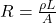where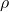is the resistivity of the material

L is the length of the conductor

A is its cross-sectional area

From the formula, we see that the resistance is proportional to the resistivity.

B)Semiconductors have resistivity values that are larger than those of insulators.  –> FALSE.

Insulators are material in which electrons cannot travel freely, so they do not conduct electricity well, and so their resistivity is very high. On the other hand, semiconductor can conduct electricity (electrons are able to move), so their resistivity is lower than insulators.

C)The resistance of a conductor is proportional to the conductivity of the material of which the conductor is composed.  –> FALSE.

The resistance of a conductor (R) is actually inversely proportional to the conductivity of the material: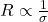where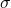is the conductivity.

D)A material that obeys Ohm’s law reasonably well is called an ohmic conductor or a linear conductor  –> TRUE.

For ohmic conductors, the relationship between voltage and current is linear, and it is given by Ohm’s law: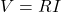where V is the voltage, I the current, and R the  resistance.

E)Good conductors of electricity have larger conductivity values than insulators. –> TRUE.

Conductivity is the reciprocal of resistance: the higher the conductivity, the better the material conducts electricity. So, good conductors have high conductivity, while insulators (which do not conduct electricity) have high resistance and low conductivity.